# Thermal Design Heat Transfer Temperature Measurement The prevalence

• Slides: 36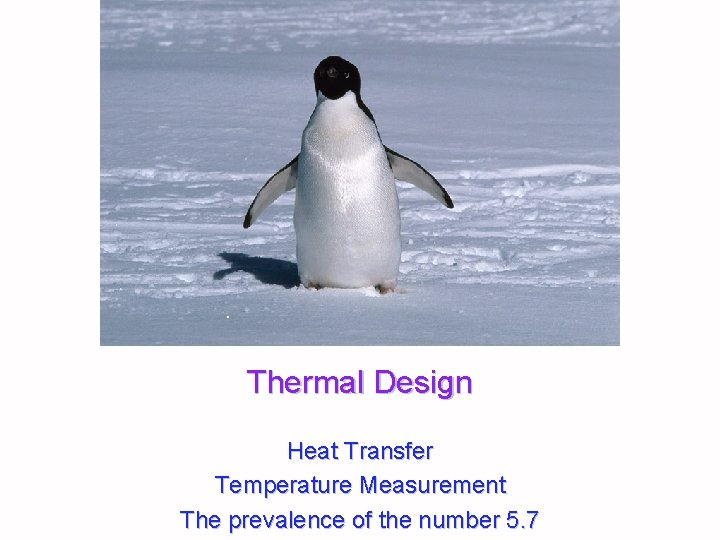Thermal Design Heat Transfer Temperature Measurement The prevalence of the number 5. 7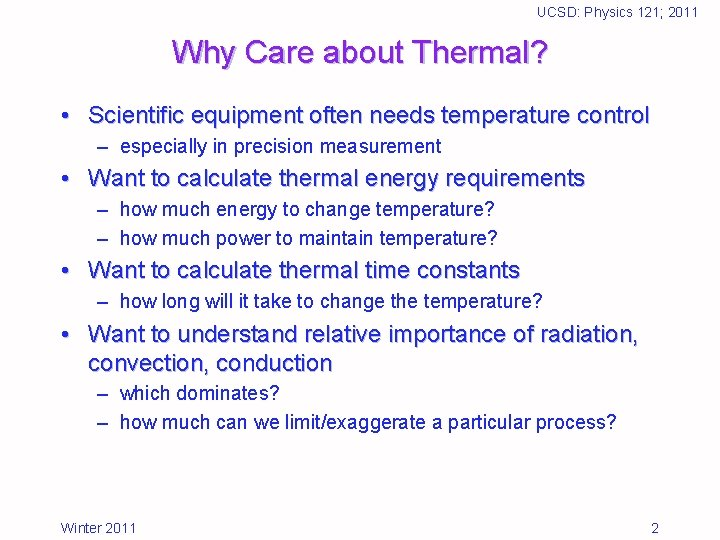UCSD: Physics 121; 2011 Why Care about Thermal? • Scientific equipment often needs temperature control – especially in precision measurement • Want to calculate thermal energy requirements – how much energy to change temperature? – how much power to maintain temperature? • Want to calculate thermal time constants – how long will it take to change the temperature? • Want to understand relative importance of radiation, convection, conduction – which dominates? – how much can we limit/exaggerate a particular process? Winter 2011 2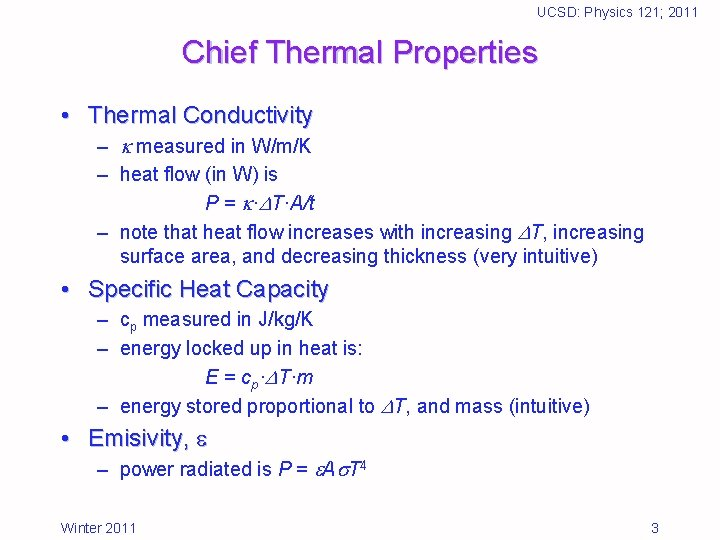UCSD: Physics 121; 2011 Chief Thermal Properties • Thermal Conductivity – measured in W/m/K – heat flow (in W) is P = · T·A/t – note that heat flow increases with increasing T, increasing surface area, and decreasing thickness (very intuitive) • Specific Heat Capacity – cp measured in J/kg/K – energy locked up in heat is: E = cp· T·m – energy stored proportional to T, and mass (intuitive) • Emisivity, – power radiated is P = A T 4 Winter 2011 3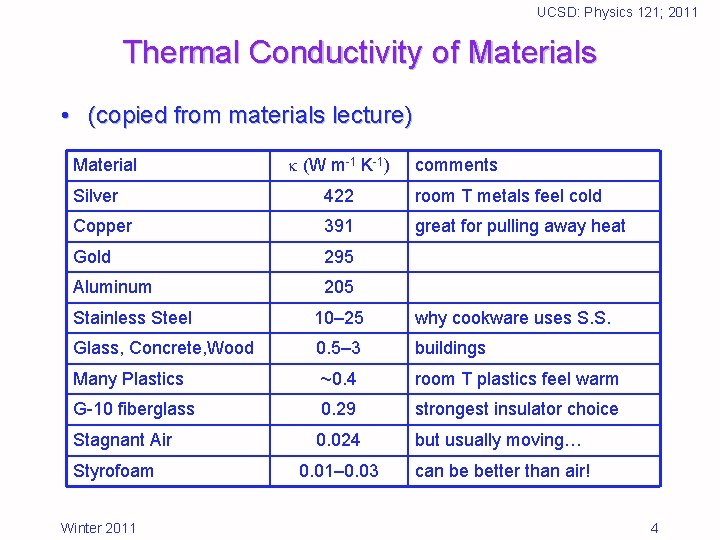UCSD: Physics 121; 2011 Thermal Conductivity of Materials • (copied from materials lecture) Material (W m-1 K-1) comments Silver 422 room T metals feel cold Copper 391 great for pulling away heat Gold 295 Aluminum 205 Stainless Steel 10– 25 why cookware uses S. S. Glass, Concrete, Wood 0. 5– 3 buildings Many Plastics ~0. 4 room T plastics feel warm G-10 fiberglass 0. 29 strongest insulator choice Stagnant Air 0. 024 but usually moving… 0. 01– 0. 03 can be better than air! Styrofoam Winter 2011 4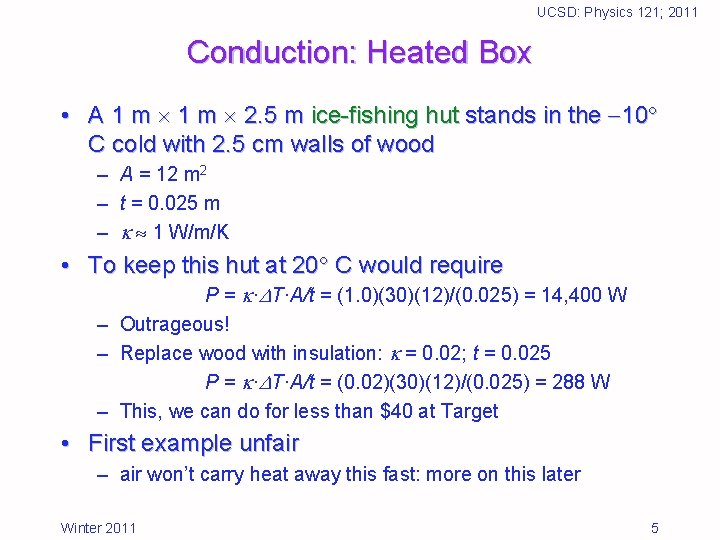UCSD: Physics 121; 2011 Conduction: Heated Box • A 1 m 2. 5 m ice-fishing hut stands in the 10 C cold with 2. 5 cm walls of wood – A = 12 m 2 – t = 0. 025 m – 1 W/m/K • To keep this hut at 20 C would require P = · T·A/t = (1. 0)(30)(12)/(0. 025) = 14, 400 W – Outrageous! – Replace wood with insulation: = 0. 02; t = 0. 025 P = · T·A/t = (0. 02)(30)(12)/(0. 025) = 288 W – This, we can do for less than \$40 at Target • First example unfair – air won’t carry heat away this fast: more on this later Winter 2011 5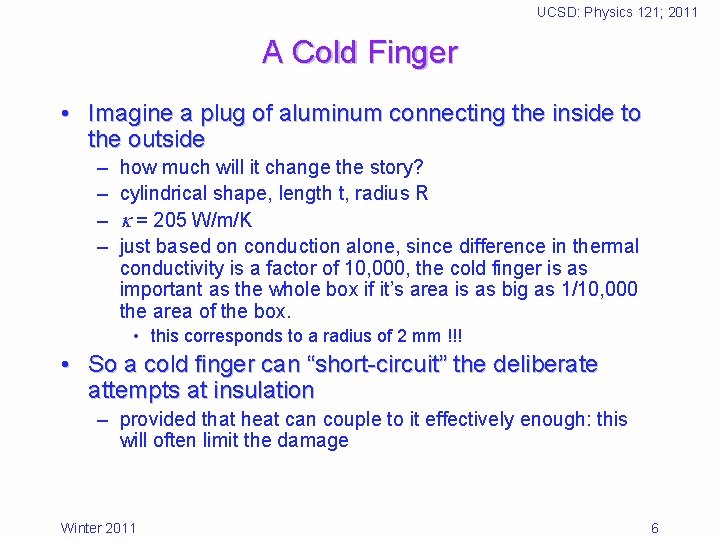UCSD: Physics 121; 2011 A Cold Finger • Imagine a plug of aluminum connecting the inside to the outside – – how much will it change the story? cylindrical shape, length t, radius R = 205 W/m/K just based on conduction alone, since difference in thermal conductivity is a factor of 10, 000, the cold finger is as important as the whole box if it’s area is as big as 1/10, 000 the area of the box. • this corresponds to a radius of 2 mm !!! • So a cold finger can “short-circuit” the deliberate attempts at insulation – provided that heat can couple to it effectively enough: this will often limit the damage Winter 2011 6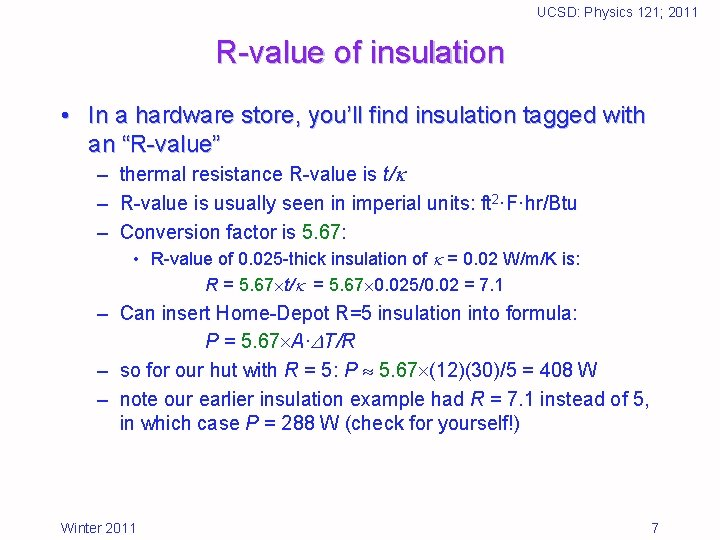UCSD: Physics 121; 2011 R-value of insulation • In a hardware store, you’ll find insulation tagged with an “R-value” – thermal resistance R-value is t/ – R-value is usually seen in imperial units: ft 2·F·hr/Btu – Conversion factor is 5. 67: • R-value of 0. 025 -thick insulation of = 0. 02 W/m/K is: R = 5. 67 t/ = 5. 67 0. 025/0. 02 = 7. 1 – Can insert Home-Depot R=5 insulation into formula: P = 5. 67 A· T/R – so for our hut with R = 5: P 5. 67 (12)(30)/5 = 408 W – note our earlier insulation example had R = 7. 1 instead of 5, in which case P = 288 W (check for yourself!) Winter 2011 7UCSD: Physics 121; 2011 Wikipedia on R-values: • Note that these examples use the non-SI definition and are per inch. Vacuum insulated panel has the highest R-value of (approximately 45 in English units) for flat, Aerogel has the next highest R-value 10, followed by isocyanurate and phenolic foam insulations with, 8. 3 and 7, respectively. They are followed closely by polyurethane and polystyrene insulation at roughly R– 6 and R– 5. Loose cellulose, fiberglass both blown and in batts, and rock wool both blown and in batts all possess an R-value of roughly 3. Straw bales perform at about R– 1. 45. Snow is roughly R– 1. • Absolutely still air has an R-value of about 5 but this has little practical use: Spaces of one centimeter or greater will allow air to circulate, convecting heat and greatly reducing the insulating value to roughly R– 1 Winter 2011 8UCSD: Physics 121; 2011 Convective Heat Exchange • Air (or any fluid) can pull away heat by physically transporting it – really conduction into fluid accompanied by motion of fluid – full, rigorous, treatment beyond scope of this class • General behavior: power convected = P = h· T·A – A is area, T is temperature difference between surface and bath – h is the convection coefficient, units: W/K/m 2 – still air has h 2– 5 W/K/m 2 • higher when T is higher: self-driven convective cells • note that h = 5. 67 is equivalent to R = 1 – gentle breeze may have h 5 – 10 W/K/m 2 – forced air may be several times larger (h 10– 50) Winter 2011 9UCSD: Physics 121; 2011 Convection Examples • Standing unclothed in a 20 C light breeze – – h 5 W/K/m 2 T = 17 C A 1 m 2 P (5)(17)(1) = 85 W • Our hut from before: – – h 5 W/K/m 2 T = 30 C (if the skin is at the hot temperature) A 12 m 2 P (5)(30)(12) = 1800 W Winter 2011 10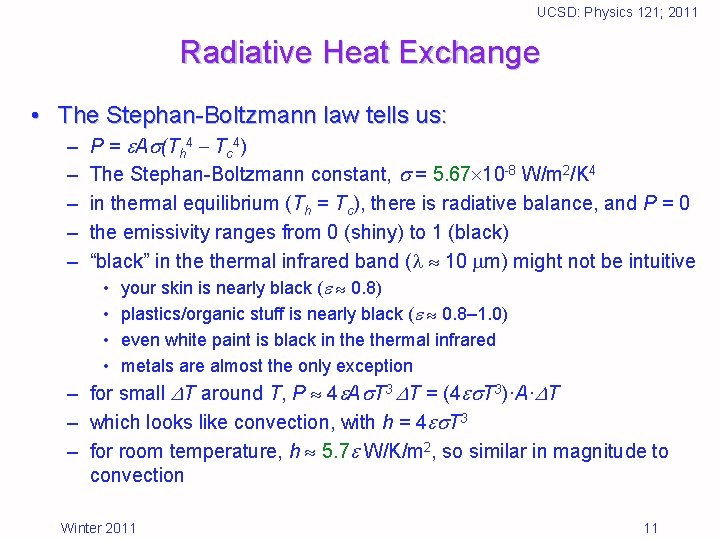UCSD: Physics 121; 2011 Radiative Heat Exchange • The Stephan-Boltzmann law tells us: – – – P = A (Th 4 Tc 4) The Stephan-Boltzmann constant, = 5. 67 10 -8 W/m 2/K 4 in thermal equilibrium (Th = Tc), there is radiative balance, and P = 0 the emissivity ranges from 0 (shiny) to 1 (black) “black” in thermal infrared band ( 10 m) might not be intuitive • your skin is nearly black ( 0. 8) • plastics/organic stuff is nearly black ( 0. 8– 1. 0) • even white paint is black in thermal infrared • metals are almost the only exception – for small T around T, P 4 A T 3 T = (4 T 3)·A· T – which looks like convection, with h = 4 T 3 – for room temperature, h 5. 7 W/K/m 2, so similar in magnitude to convection Winter 2011 11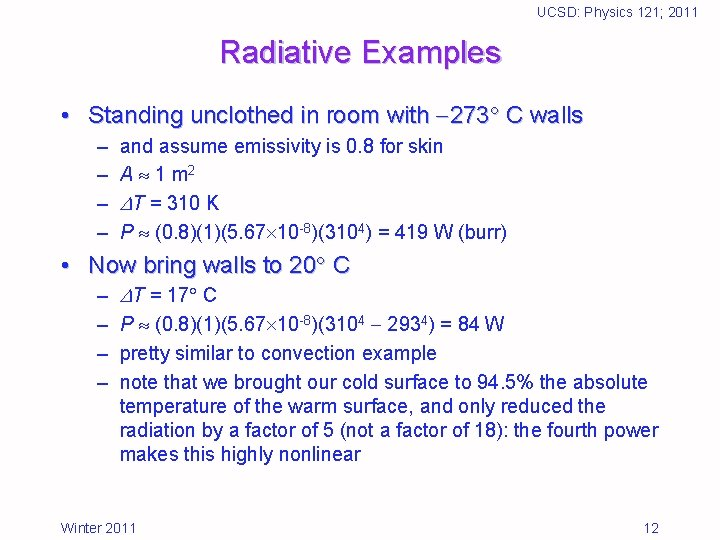UCSD: Physics 121; 2011 Radiative Examples • Standing unclothed in room with 273 C walls – – and assume emissivity is 0. 8 for skin A 1 m 2 T = 310 K P (0. 8)(1)(5. 67 10 -8)(3104) = 419 W (burr) • Now bring walls to 20 C – – T = 17 C P (0. 8)(1)(5. 67 10 -8)(3104 2934) = 84 W pretty similar to convection example note that we brought our cold surface to 94. 5% the absolute temperature of the warm surface, and only reduced the radiation by a factor of 5 (not a factor of 18): the fourth power makes this highly nonlinear Winter 2011 12UCSD: Physics 121; 2011 Combined Problems • Two-layer insulation – must compute temperature at interface • Conduction plus Convection – skin temperature must be solved • Conduction plus Radiation – skin temperature must be solved • The whole enchilada – conduction, convection, radiation Winter 2011 13UCSD: Physics 121; 2011 Two-Layer insulation • Let’s take our ice-fishing hut and add insulation instead of replacing the wood with insulation – – – each still has thickness 0. 025 m; and surface area 12 m 2 Now have three temperatures: Tin = 20 , Tmid, Tout = 10 Flow through first is: P 1 = 1·(Tin Tmid)·A 1/t 1 Flow through second is: P 2 = 2·(Tmid Tout)·A 2/t 2 In thermal equilibrium, must have P 1 = P 2 • else energy is building up or coming from nowhere – We know everything but Tmid, which we easily solve for: Tmid( 1 A 1/t 1 + 2 A 2/t 2 ) = 2 A 2 Tin/t 2 + 2 A 2 Tout/t 2 – find Tmid = 9. 412 or Tmid = 19. 412 depending on which is interior or exterior – heat flow is 282 W (compare to 288 W before: wood hardly matters) Winter 2011 14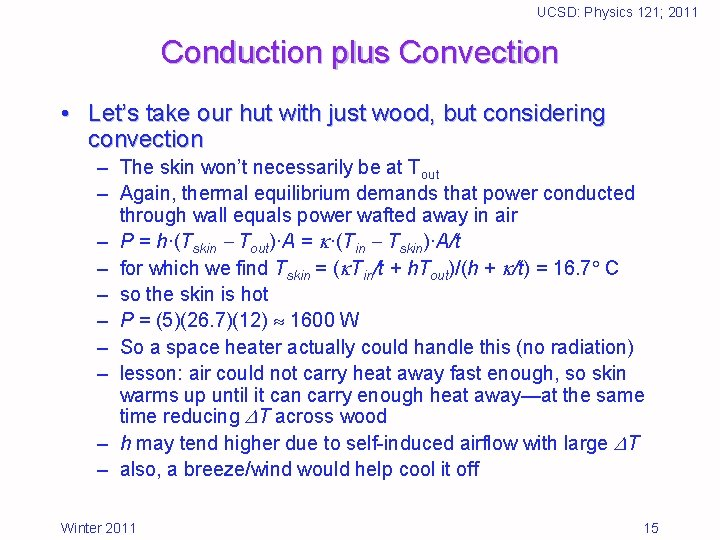UCSD: Physics 121; 2011 Conduction plus Convection • Let’s take our hut with just wood, but considering convection – The skin won’t necessarily be at Tout – Again, thermal equilibrium demands that power conducted through wall equals power wafted away in air – P = h·(Tskin Tout)·A = ·(Tin Tskin)·A/t – for which we find Tskin = ( Tin/t + h. Tout)/(h + /t) = 16. 7 C – so the skin is hot – P = (5)(26. 7)(12) 1600 W – So a space heater actually could handle this (no radiation) – lesson: air could not carry heat away fast enough, so skin warms up until it can carry enough heat away—at the same time reducing T across wood – h may tend higher due to self-induced airflow with large T – also, a breeze/wind would help cool it off Winter 2011 15UCSD: Physics 121; 2011 Convection plus Radiation • How warm should a room be to stand comfortably with no clothes? – assume you can put out P = 100 W metabolic power • 2000 kcal/day = 8, 368, 000 J in 86400 sec 100 W – – – – P = h·(Tskin Tout)·A + A (Tskin 4 Tout 4) (h. A + 4 A T 3) T with emissivity = 0. 8, T = 293 K 100 = ((5)(1) + 4. 56) T T = 10. 5 so the room is about 310 10. 5 = 299. 5 K = 26. 5 C = 80 F iterating (using T = 299. 5); 4. 56 4. 87; T 10. 1 assumes skin is full internal body temperature • some conduction in skin reduces skin temperature • so could tolerate slightly cooler Winter 2011 16UCSD: Physics 121; 2011 The whole enchilada • Let’s take a cubic box with a heat source inside and consider all heat transfers – – – – P = 1 W internal source inside length = 10 cm thickness = 2. 5 cm R-value = 5 so 5. 67 t/ = 5 = 0. 028 W/m/K effective conductive area is 12. 5 cm cube Ac = 0. 09375 m 2 external (radiative, convective) area is 15 cm cube Aext = 0. 135 m 2 – assume h = 5 W/K/m 2, = 0. 8, Text = 293 K – assume the air inside is thoroughly mixed (perhaps 1 W source is a fan!) Winter 2011 17UCSD: Physics 121; 2011 The enchilada calculation • power generated = power conducted = power convected plus power radiated away P = ·(Tin Tskin)·Ac/t = (h. Aext + 4 Aext T 3)·(Tskin Text) – first get Tskin from convective/radiative piece – Tskin = Text + P/ (h. Aext + 4 Aext T 3) = 20 + 1. 0/(0. 675+0. 617) – Tskin = 20. 8 (barely above ambient) – now the T across the insulation is P·t/(Ac· ) = 9. 5 – so Tin = 30. 3 • Notice a few things: – radiation and convection nearly equal influence (0. 617 vs. 0. 675) – shutting off either would result in small (but measurable) change Winter 2011 18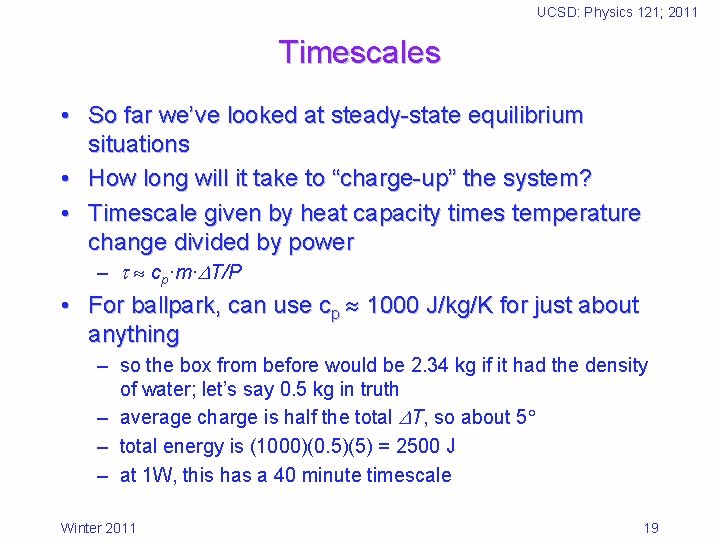UCSD: Physics 121; 2011 Timescales • So far we’ve looked at steady-state equilibrium situations • How long will it take to “charge-up” the system? • Timescale given by heat capacity times temperature change divided by power – cp·m· T/P • For ballpark, can use cp 1000 J/kg/K for just about anything – so the box from before would be 2. 34 kg if it had the density of water; let’s say 0. 5 kg in truth – average charge is half the total T, so about 5 – total energy is (1000)(0. 5)(5) = 2500 J – at 1 W, this has a 40 minute timescale Winter 2011 19UCSD: Physics 121; 2011 Heating a lump by conduction • Heating food from the outside, one relies entirely on thermal conduction/diffusion to carry heat in • Relevant parameters are: – – thermal conductivity, (how fast does heat move) (W/m/K) heat capacity, cp (how much heat does it hold) (J/kg/K) mass, m (how much stuff is there) (kg) size, R—like a radius (how far does heat have to travel) (m) • Just working off units, derive a timescale: – – – (cp/ )(m/R) 4(cp/ ) R 2 where is density, in kg/m 3: m/((4/3) R 3) m/4 R 3 faster if: cp is small, is large, R is small (these make sense) for typical food values, 6 minutes (R/1 cm)2 egg takes ten minutes, turkey takes 5 hours Winter 2011 20UCSD: Physics 121; 2011 Lab Experiment • We’ll build boxes with a heat load inside to test the ideas here • In principle, we can: – – – measure thermal conductivity of the insulation see the impact of emissivity changes see the impact of enhanced convection look for thermal gradients in the absence of circulation look at the impact of geometry on thermal state see how serious heat leaks can be • Nominal box: – 10 cm side, 1 -inch thick, about 1. 5 W (with fan) Winter 2011 21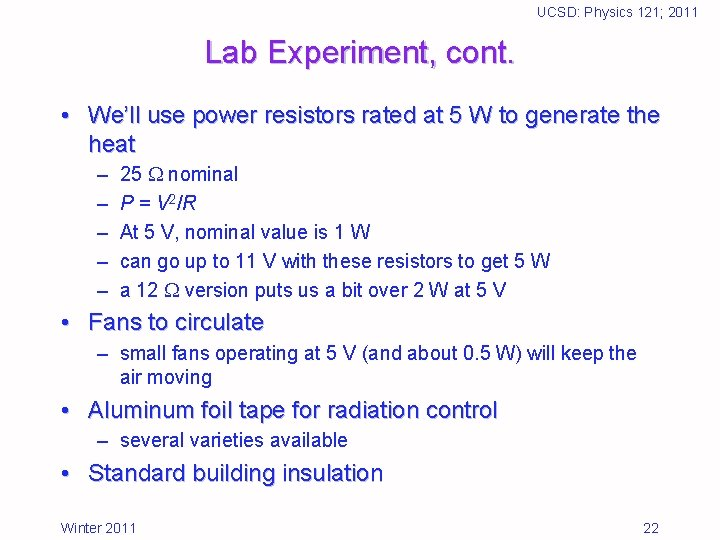UCSD: Physics 121; 2011 Lab Experiment, cont. • We’ll use power resistors rated at 5 W to generate the heat – – – 25 nominal P = V 2/R At 5 V, nominal value is 1 W can go up to 11 V with these resistors to get 5 W a 12 version puts us a bit over 2 W at 5 V • Fans to circulate – small fans operating at 5 V (and about 0. 5 W) will keep the air moving • Aluminum foil tape for radiation control – several varieties available • Standard building insulation Winter 2011 22UCSD: Physics 121; 2011 Lab Experimental Suite experiment R int. airflow ext. airflow int. foil ext. foil geom. A (control) 25 1 fan none no no 10 cm cube B (ext. convec) 25 1 fan no no 10 cm cube C (ext. radiation) 25 1 fan none no yes 10 cm cube D (ext. conv/rad) 25 1 fan no yes 10 cm cube E (gradients) 25 none no no 10 cm cube F (int. radiation) 25 1 fan none yes no 10 cm cube G (radiation) 25 1 fan none yes 10 cm cube H (more power) 12 1 fan none no no 10 cm cube I (larger area) 12 2 fans none no no 17. 5 cm cube J (area and thick. ) 12 2 fans none no no 17. 5 cm cube Winter 2011 23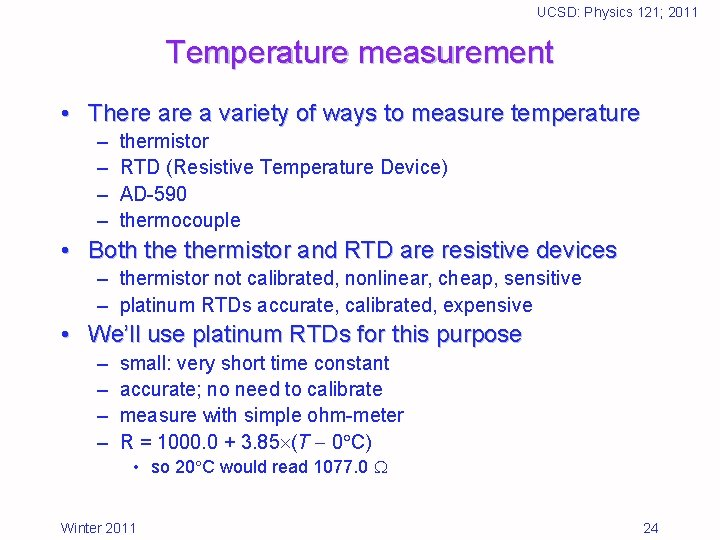UCSD: Physics 121; 2011 Temperature measurement • There a variety of ways to measure temperature – – thermistor RTD (Resistive Temperature Device) AD-590 thermocouple • Both thermistor and RTD are resistive devices – thermistor not calibrated, nonlinear, cheap, sensitive – platinum RTDs accurate, calibrated, expensive • We’ll use platinum RTDs for this purpose – – small: very short time constant accurate; no need to calibrate measure with simple ohm-meter R = 1000. 0 + 3. 85 (T 0 C) • so 20 C would read 1077. 0 Winter 2011 24UCSD: Physics 121; 2011 Random Notes • Rig fan and resistor in parallel, running off 5 V – fan can accept range: 4. 5– 5. 5 V – if you want independent control, don’t rig together • Use power supply current reading (plus voltage) to ascertain power (P = IV) being delivered into box • Make sure all RTDs read same thing on block of thermally stabilized chunk of metal – account for any offset in analysis • Don’t let foil extend to outside as a cold finger • Make sure you have no air gaps: tape inside and out of seams – but need to leave top accessible – nice to tape fan to top (avoid heat buildup here) – can hang resistor, RTD from top as well (easy to assemble) Winter 2011 25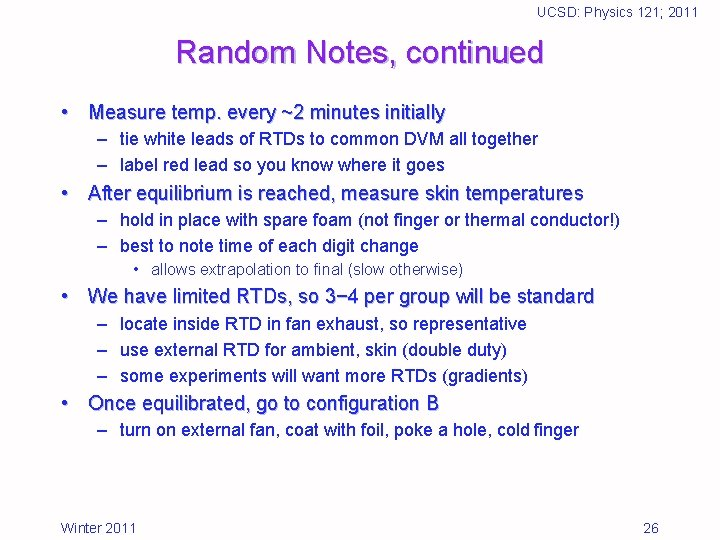UCSD: Physics 121; 2011 Random Notes, continued • Measure temp. every ~2 minutes initially – tie white leads of RTDs to common DVM all together – label red lead so you know where it goes • After equilibrium is reached, measure skin temperatures – hold in place with spare foam (not finger or thermal conductor!) – best to note time of each digit change • allows extrapolation to final (slow otherwise) • We have limited RTDs, so 3− 4 per group will be standard – locate inside RTD in fan exhaust, so representative – use external RTD for ambient, skin (double duty) – some experiments will want more RTDs (gradients) • Once equilibrated, go to configuration B – turn on external fan, coat with foil, poke a hole, cold finger Winter 2011 26UCSD: Physics 121; 2011 Random Notes, continued • Send your data points to me via e-mail so I can present the amalgam of results to the class – use format: hh: mm RTD 1 RTD 2 RTD 3 etc. – example: 11: 43 1088 1155 1152 1228 – include a description of what each column represents • Also include basic setup and changes in e-mail so I know what I’m plotting • Also include in the message temperatures you measure only once, or occasionally (like skin temp. ) • I’ll make the data available for all to access for the write-ups Winter 2011 27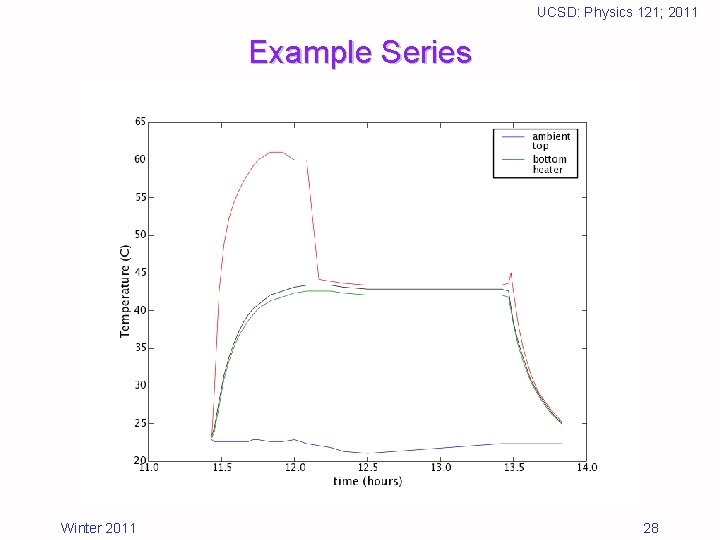UCSD: Physics 121; 2011 Example Series Winter 2011 28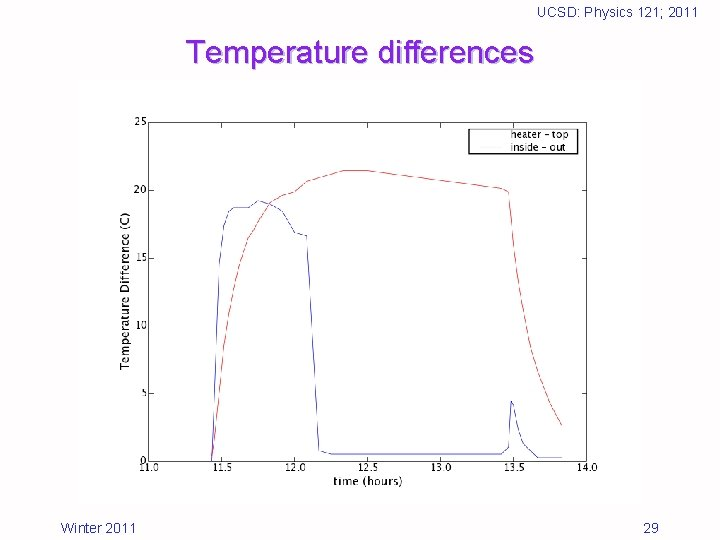UCSD: Physics 121; 2011 Temperature differences Winter 2011 29UCSD: Physics 121; 2011 References and Assignment • Useful text: – Introduction to Heat Transfer: Incropera & De. Witt • Reading in text: – Chapter 8 (7 in 3 rd ed. ) reading assignment: check web page for details Winter 2011 30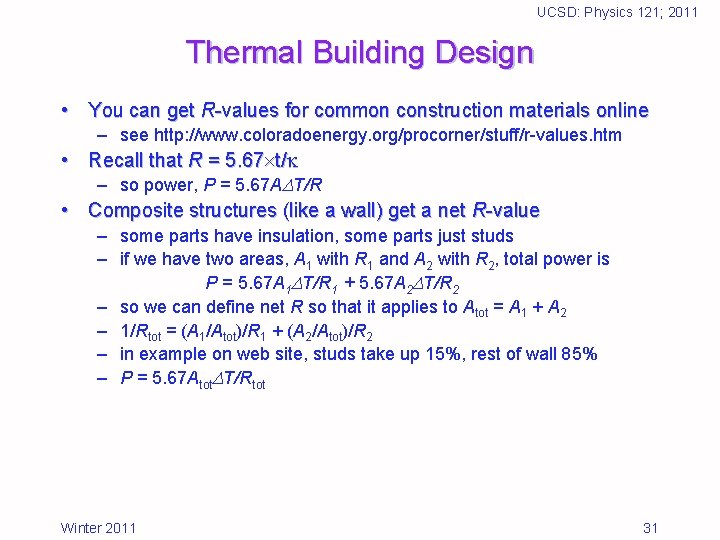UCSD: Physics 121; 2011 Thermal Building Design • You can get R-values for common construction materials online – see http: //www. coloradoenergy. org/procorner/stuff/r-values. htm • Recall that R = 5. 67 t/ – so power, P = 5. 67 A T/R • Composite structures (like a wall) get a net R-value – some parts have insulation, some parts just studs – if we have two areas, A 1 with R 1 and A 2 with R 2, total power is P = 5. 67 A 1 T/R 1 + 5. 67 A 2 T/R 2 – so we can define net R so that it applies to Atot = A 1 + A 2 – 1/Rtot = (A 1/Atot)/R 1 + (A 2/Atot)/R 2 – in example on web site, studs take up 15%, rest of wall 85% – P = 5. 67 Atot T/Rtot Winter 2011 31UCSD: Physics 121; 2011 Handling External Flow as R-value • On the materials site, they assign R-values to the air “layer” up against the walls – outside skin R = 0. 17 – inside skin R = 0. 68 • This accounts for both convection and radiation. How? – recall that power through the walls has to equal the power convected and radiated P = 5. 67 A(Tin Tskin)/R = hconv. A(Tskin Tout) + hrad. A(Tskin Tout) P = 5. 67 A(Tin Tskin)/R = heff. A(Tskin Tout) – where hrad 4 T 3, and heff = hconv + hrad • We can solve this for Tskin, to find Tskin = (5. 67 Tin/R + heff. Tout)/(5. 67/R +heff) Winter 2011 32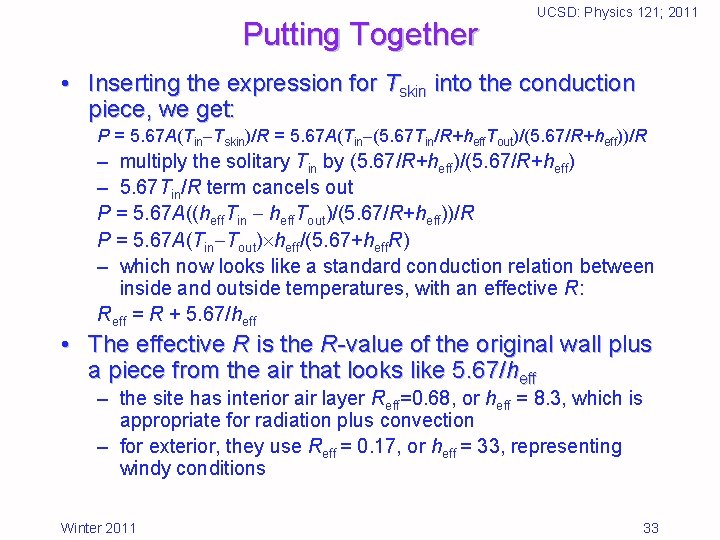Putting Together UCSD: Physics 121; 2011 • Inserting the expression for Tskin into the conduction piece, we get: P = 5. 67 A(Tin Tskin)/R = 5. 67 A(Tin (5. 67 Tin/R+heff. Tout)/(5. 67/R+heff))/R – multiply the solitary Tin by (5. 67/R+heff)/(5. 67/R+heff) – 5. 67 Tin/R term cancels out P = 5. 67 A((heff. Tin heff. Tout)/(5. 67/R+heff))/R P = 5. 67 A(Tin Tout) heff/(5. 67+heff. R) – which now looks like a standard conduction relation between inside and outside temperatures, with an effective R: Reff = R + 5. 67/heff • The effective R is the R-value of the original wall plus a piece from the air that looks like 5. 67/heff – the site has interior air layer Reff=0. 68, or heff = 8. 3, which is appropriate for radiation plus convection – for exterior, they use Reff = 0. 17, or heff = 33, representing windy conditions Winter 2011 33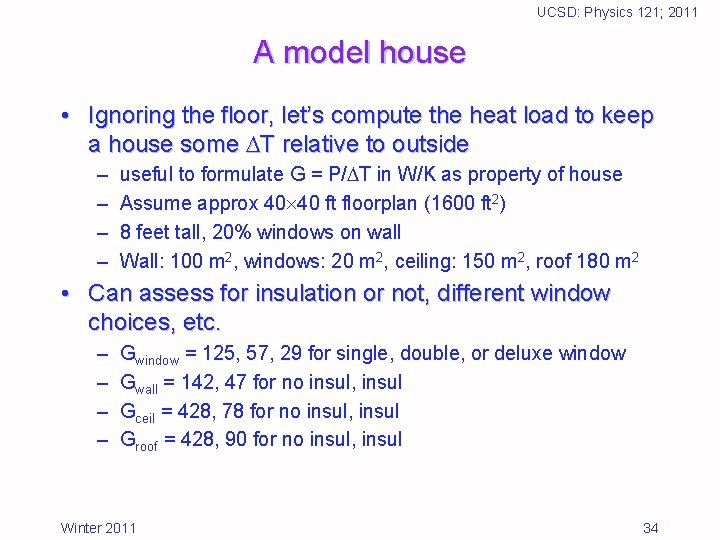UCSD: Physics 121; 2011 A model house • Ignoring the floor, let’s compute the heat load to keep a house some T relative to outside – – useful to formulate G = P/ T in W/K as property of house Assume approx 40 40 ft floorplan (1600 ft 2) 8 feet tall, 20% windows on wall Wall: 100 m 2, windows: 20 m 2, ceiling: 150 m 2, roof 180 m 2 • Can assess for insulation or not, different window choices, etc. – – Gwindow = 125, 57, 29 for single, double, or deluxe window Gwall = 142, 47 for no insul, insul Gceil = 428, 78 for no insul, insul Groof = 428, 90 for no insul, insul Winter 2011 34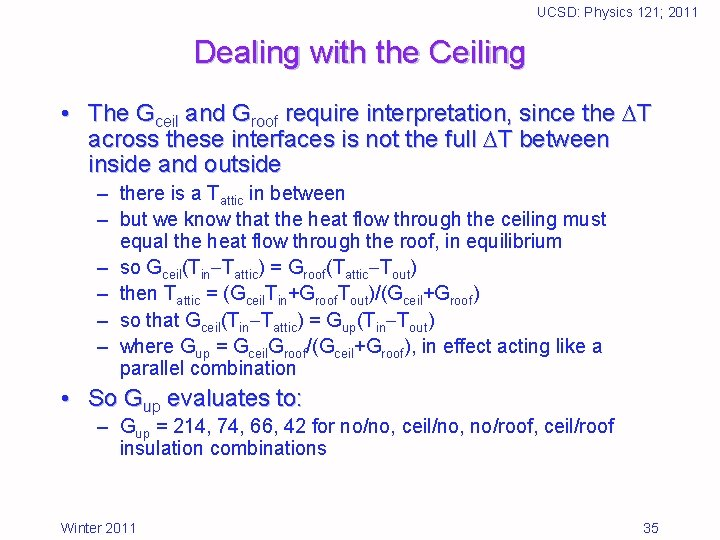UCSD: Physics 121; 2011 Dealing with the Ceiling • The Gceil and Groof require interpretation, since the T across these interfaces is not the full T between inside and outside – there is a Tattic in between – but we know that the heat flow through the ceiling must equal the heat flow through the roof, in equilibrium – so Gceil(Tin Tattic) = Groof(Tattic Tout) – then Tattic = (Gceil. Tin+Groof. Tout)/(Gceil+Groof) – so that Gceil(Tin Tattic) = Gup(Tin Tout) – where Gup = Gceil. Groof/(Gceil+Groof), in effect acting like a parallel combination • So Gup evaluates to: – Gup = 214, 74, 66, 42 for no/no, ceil/no, no/roof, ceil/roof insulation combinations Winter 2011 35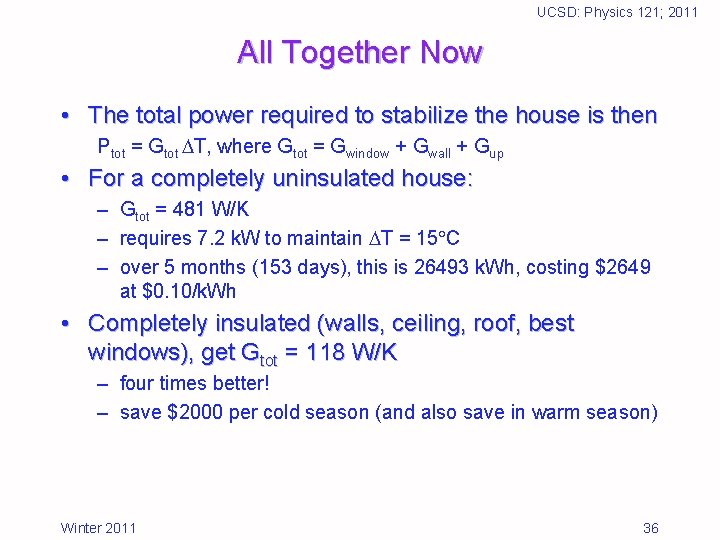UCSD: Physics 121; 2011 All Together Now • The total power required to stabilize the house is then Ptot = Gtot T, where Gtot = Gwindow + Gwall + Gup • For a completely uninsulated house: – Gtot = 481 W/K – requires 7. 2 k. W to maintain T = 15 C – over 5 months (153 days), this is 26493 k. Wh, costing \$2649 at \$0. 10/k. Wh • Completely insulated (walls, ceiling, roof, best windows), get Gtot = 118 W/K – four times better! – save \$2000 per cold season (and also save in warm season) Winter 2011 36